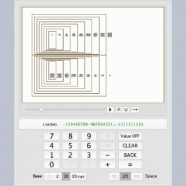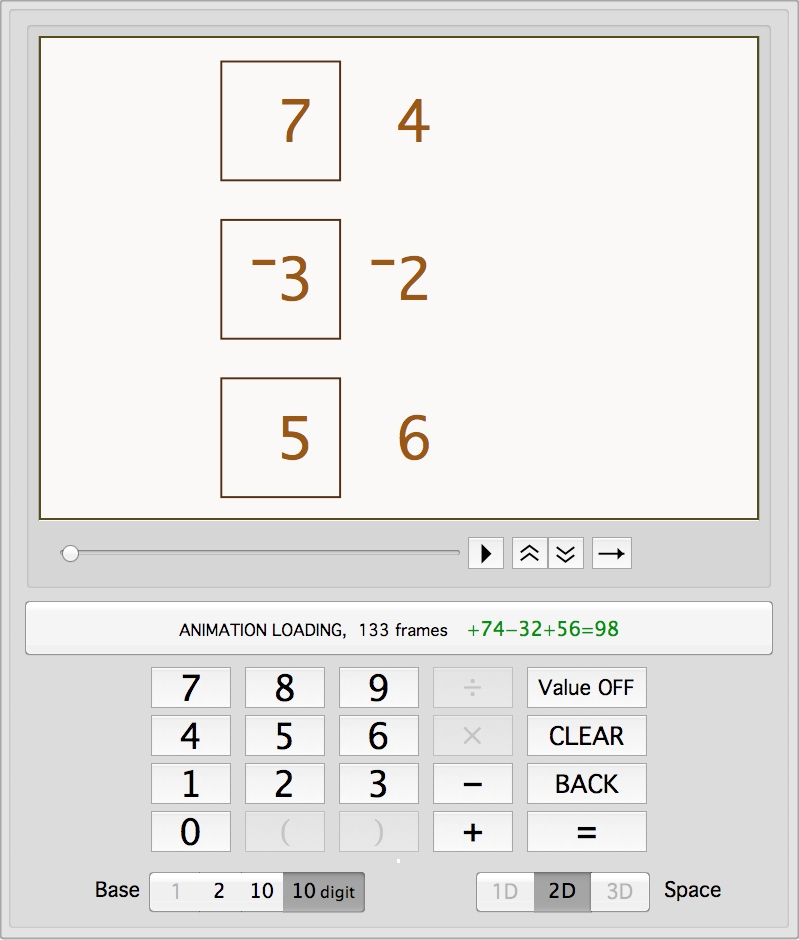# Iconic CalculatorThe Iconic Arithmetic Calculator illustrates addition and subtraction of container numbers. It works just like a regular hand calculator, except that calculation of the results is shown dynamically on the display screen. Iconic numbers show the process of calculation, so they are particularly useful for students and others learning how arithmetic works. The Image shows the Calculator in the process of adding two nine-digit numbers in decimal unit mode. The Container Numbers page shows how to read container numbers, animates the Rules of Iconic Arithmetic, and provides animated examples of addition, subtraction, multiplication, and division. The Depth-value Notation page describes how containers improve upon our universal place-value notation for numbers. The Iconic Algebra page describes the theory of container numbers.

During the design and development of software, many documents that describe how the software works are constructed. If you are interestes in more technical details, here are three development documents. The overview and descriptive details about the design of the calculator and the number systems it implements are in the technical paper Design and Implementation of a Boundary Arithmetic Calculator. The pattern rules that determine how the calculator works, together with example algorithms, are described in Whole Number Arithmetic (+, –, x, ÷) in the Iconic Calculator. Finally, the complete animation of the sume 18–9+18 is described frame-by-frame in Graphic Engine Input/Output.

The Iconic Calculator is written in the Mathematica language. It is fully functional, although multiplication and division are not yet implemented (as of July 2013). Unfortunately the Calculator is not available online at this time.

The Calculator does arithmetic very differently than we are taught in school.  It is iconic rather than symbolic, so that each step in the computation is a physical activity that is displayed as the computation occurs. All digit operations occur at the same time rather than sequentially. Addition and subtraction can occur together during the same computation. Many numbers can be added all at the same time. Most of the conventional rules of arithmetic are shown to be not only unnecessary but also limiting to both concept and calculation.  For example, our common place-value numbers are easy to read, they are hard to work with and require excessive mental energy.

The Iconic Calculator provides a choice of preferred number system.  It displays four different number systems:

unary (base-1), the unit-ensemble or tally system with no grouping of units.

binary (base-2), the system used by computers, with only two digits.

decimal with units (base-10), the decimal system with unit-ensembles in place of digits.

decimal with digits (base-10), our usual number system with ten digits.

The unary or tally system does not require digits so there are no number facts (such as 3+4=7) to memorize. Tallies also do not use grouping, so there are no place-values to keep track of. The difficulty with tallies is that large collections of units are hard to count. The binary system clearly illustrates how iconic arithmetic works. Since there is no iconic zero, for example, the two signs (such as 0 and 1) of conventional binary numbers reduces to one sign, the presence or absence of a unit (). Again the difficulty is that it is hard to read results when they are not expressed in the decimal notation we have learned.  The decimal with units system is similar to what we currently use, but with unit-ensembles in place of digits.   Each digit is represented by a corresponding collection of units. These units combine following the Additive Principle, and only need to be grouped when ten or more units are inside any one container.  Number facts are not needed and tracking the position of digits is not necessary.  The decimal with digits system is our common numbering system, but with depth-value rather than place-value keeping track of magnitude.  Digits can be used within iconic arithmetic at the cost of having to know about twenty number facts.

Here is an image of the Iconic Arithmetic Calculator interface.  The calculation that has been typed in is 74–32+56. The display shows the initial layout of these three numbers prior to the animation of addition/subtraction.The interface provides choices for both number system and dimensionality of the display. The one-dimensional container notation is parens, which is described and used extensively on the Container Numbers page. The two-dimensional notation is the rectangular containers that are currently showing. The three-dimensional notation converts the 2D containers into physical boxes with depth. The three simplification rules for iconic arithmetic, Merging containers, Grouping units and Canceling opposites remain the same, regardless of the dimensionality or the base of the representation. Parens are good for 1D typographical display while rectangular boxes are good for sketching and for 2D visualization. 3D boxes provide a representation that can be physically manipulated, allowing arithmetic to be performed with physical objects like pots and pans. (July 2013: Dimensionality is not yet fully implemented.)

The animated display has the usual controls found in a video player: start/stop, slow/fast, forward/backward, and dynamic control using a slider. For ease of reading, the Value button lets a user position the cursor over any part of the display to pop up an information box that shows in conventional notation the current value of that location.

Internally, the operations typed into the Calculator are converted first to parens form. Iconic simplification (Merge, Group, and Cancel) is applied to the parens form, while each transformation step is stored. Once the answer has been calculated, the parens transformation sequences are given to the animation engine which builds a sequence of frames that illustrate the structural changes embodied by each transformation step. The animation is then loaded into the display panel for interactive viewing.